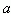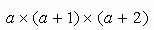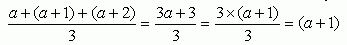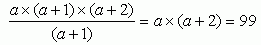Name: Sandy Who is asking: Student Level of the question: Middle Question: When I divided the product of three consecutive positive integers by their mean, I got 99. What is the smallest of the three numbers? Hi Sandy. Let's decide to call the smallest of the three numbers. Then the product of the three consecutive integers is:The mean of these numbers is their sum divided by three:That's neat - the mean of three consecutive numbers is just the second number! Now you say you get 99 if you divide the product by the mean. If we do that and then do some cancelling, we get:Now you know that if you multiply the smallest number by a number 2 larger, you'll get 99. You can take it from here! Stephen La Rocque>